# How to set the RadioButton to Checked State in C#?

• Last Updated : 30 Jun, 2019

In Windows Forms, RadioButton control is used to select a single option among the group of the options. For example, select your gender from the given list, so you will choose only one option among three options like Male or Female or Transgender. In Windows Forms, you are allowed to set a value which represents the RadioButton control is checked using the Checked Property of the RadioButton.
If the value of this property is set to true, then RadioButton is checked and if the value of this property is set to false, then RadioButton is not checked. The default value of this property is false. You can set this property in two different ways:

1. Design-Time: It is the easiest way to set a value which represents the RadioButton is checked as shown in the following steps:

• Step 1: Create a windows form as shown in the below image:
Visual Studio -> File -> New -> Project -> WindowsFormApp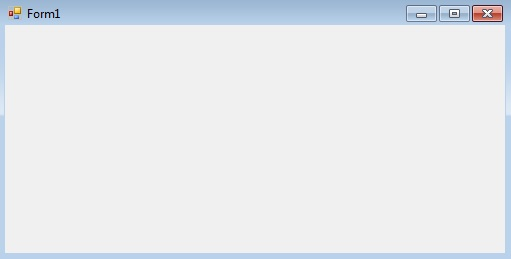• Step 2: Drag the RadioButton control from the ToolBox and drop it on the windows form. You are allowed to place a RadioButton control anywhere on the windows form according to your need.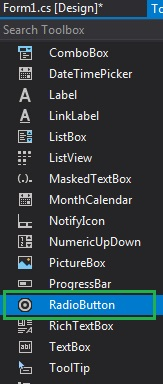• Step 3: After drag and drop you will go to the properties of the RadioButton control to set a value which represents the RadioButton control is checked.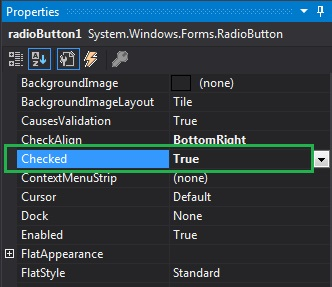Output: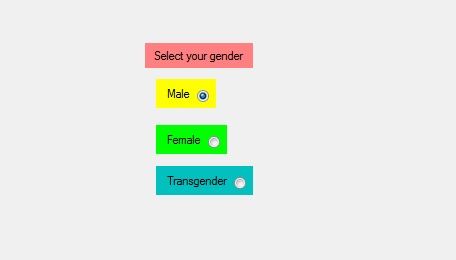2. Run-Time: It is a little bit trickier than the above method. In this method, you can set a value which represents the RadioButton control is checked programmatically with the help of given syntax:

`public bool Checked { get; set; }`

The value of this property is of System.Boolean type. The following steps show how to set the Checked property of the RadioButton dynamically:

• Step 1: Create a radio button using the RadioButton() constructor is provided by the RadioButton class.
```// Creating radio button
```
• Step 2: After creating RadioButton, set the Checked property of the RadioButton provided by the RadioButton class.
```// Setting the Checked property of the radio button
r1.Checked = true;
```
```// Add this radio button to the form
```

Example:

 `using` `System;``using` `System.Collections.Generic;``using` `System.ComponentModel;``using` `System.Data;``using` `System.Drawing;``using` `System.Linq;``using` `System.Text;``using` `System.Threading.Tasks;``using` `System.Windows.Forms;`` ` `namespace` `WindowsFormsApp24 {`` ` `public` `partial` `class` `Form1 : Form {`` ` `    ``public` `Form1()``    ``{``        ``InitializeComponent();``    ``}`` ` `    ``private` `void` `RadioButton2_CheckedChanged(``object` `sender, ``                                               ``EventArgs e)``    ``{``    ``}`` ` `    ``private` `void` `Form1_Load(``object` `sender, EventArgs e)``    ``{``        ``// Creating and setting label``        ``Label l = ``new` `Label();``        ``l.AutoSize = ``true``;``        ``l.Location = ``new` `Point(176, 40);``        ``l.Text = ``"Select Post"``;`` ` `        ``// Adding this label to the form``        ``this``.Controls.Add(l);`` ` `        ``// Creating and setting the ``        ``// properties of the RadioButton``        ``RadioButton r1 = ``new` `RadioButton();``        ``r1.AutoSize = ``true``;``        ``r1.Text = ``"Intern"``;``        ``r1.Location = ``new` `Point(286, 40);``        ``r1.Checked = ``true``;`` ` `        ``// Adding this label to the form``        ``this``.Controls.Add(r1);`` ` `        ``// Creating and setting the``        ``// properties of the RadioButton``        ``RadioButton r2 = ``new` `RadioButton();``        ``r2.AutoSize = ``true``;``        ``r2.Text = ``"Team Leader"``;``        ``r2.Location = ``new` `Point(356, 40);``        ``r2.Checked = ``false``;`` ` `        ``// Adding this label to the form``        ``this``.Controls.Add(r2);`` ` `        ``// Creating and setting the ``        ``// properties of the RadioButton``        ``RadioButton r3 = ``new` `RadioButton();``        ``r3.AutoSize = ``true``;``        ``r3.Text = ``"Software Engineer"``;``        ``r3.Location = ``new` `Point(470, 40);``        ``r3.Checked = ``true``;`` ` `        ``// Adding this label to the form``        ``this``.Controls.Add(r3);``    ``}``}``}`

Output:

Before setting the Checked property: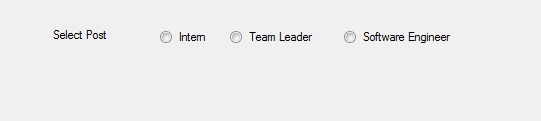After setting the Checked property: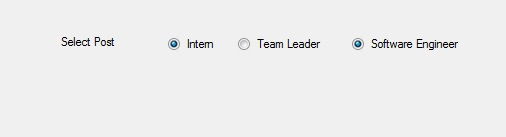My Personal Notes arrow_drop_up